# Introduction

This file contains some examples of the functions related to the DI and cellHandler routines.

``````library("cellWise")
``````

# Artificial data

In this example we consider an artificial dataset with cellwise outliers. First we construct a correlation matrix and then use it to generate the data.

``````d     <- 10
mu    <- rep(0, 10)
Sigma <- generateCorMat(d = d, corrType = "A09")

n      <- 100 # number of observations
outlierType   <- "cellwiseStructured" # type of cellwise outliers
perout <- 0.2 # percentage of outliers
gamma  <- 5 # how far the outliers are from the center

data  <- generateData(n, d, mu, Sigma, perout,
gamma, outlierType, seed = 1)
X <- data\$X
pairs(X)
``````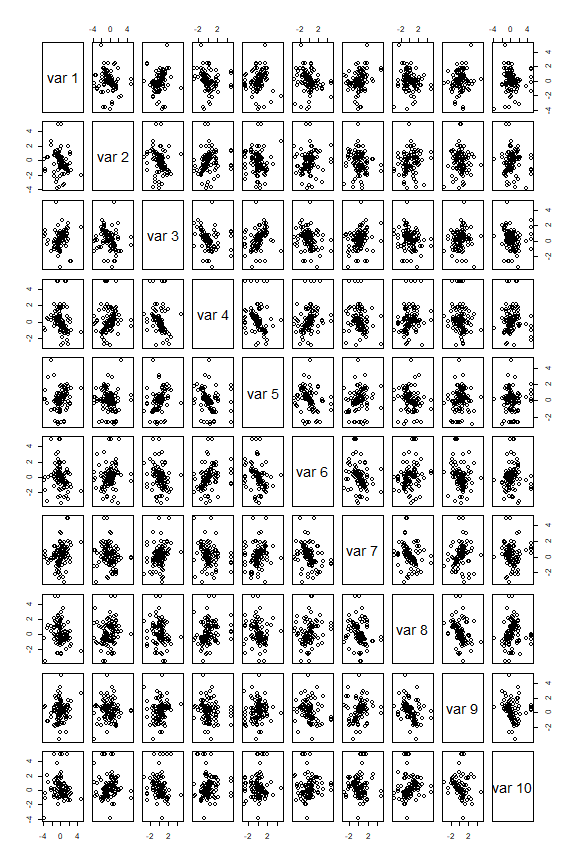``````# we clearly see some marginal outliers, but also some more tricky ones.
``````

Now we run the DI algorithm to detect cellwise outliers

``````tic = Sys.time()
DI.out = DI(X)
``````
``````##
##  The input data has 100 rows and 10 columns.
``````
``````toc = Sys.time(); toc - tic
``````
``````## Time difference of 0.1151021 secs
``````
``````DI.out\$nits
``````
``````##  4
``````
``````# the algorithm converges in 4 iterations and takes under 1 second

flaggedCells <- DI.out\$indcells # indices of the flagged Cells
length(intersect(data\$indcells, flaggedCells))
``````
``````##  159
``````
``````# 159 of the 200 cells are flagged

# We can now compare this with the marginal flagging of outliers.
locScale.out <- estLocScale(X) # robust location and scale of X
Z <- scale(X, locScale.out\$loc, locScale.out\$scale)
flaggedCells_marginal <- which(abs(Z) >  sqrt(qchisq(p = 0.99, df = 1)))
length(intersect(data\$indcells, flaggedCells_marginal))
``````
``````##  61
``````
``````# only 61 of the 200 cells are flagged

cellMap(X, DI.out\$Zres, indcells = flaggedCells,
columnlabels = 1:10,
rowlabels = 1:100,
mTitle = "cellHandler",
rowtitle = "",
columntitle = "",
sizetitles = 2,
drawCircles = F)
``````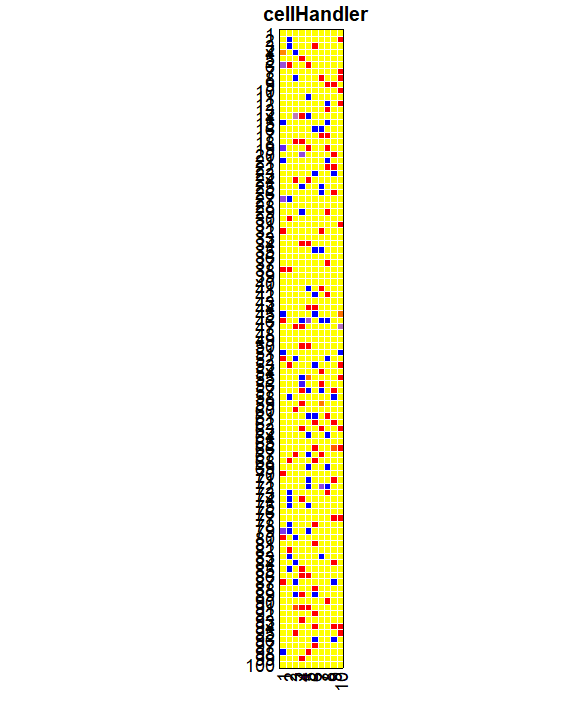``````cellMap(X, Z, indcells = flaggedCells_marginal,
columnlabels = 1:10,
rowlabels = 1:100,
mTitle = "marginal analysis",
rowtitle = "",
columntitle = "",
sizetitles = 2,
drawCircles = F)
``````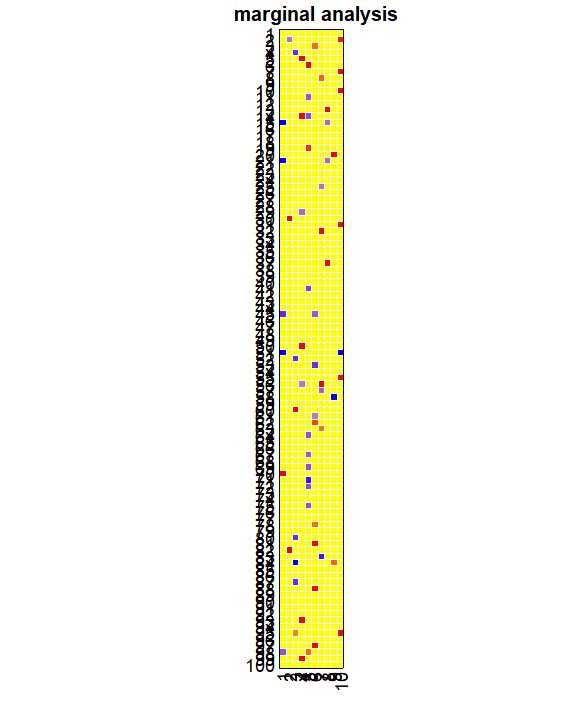# VOC data

We first load an inspect the data.

``````data("data_VOC")
# ?VOC

# The first 16 variables are the VOCs, the last 3 are:
# SMD460: How many people who live here smoke tobacco?
# SMD470: How many people smoke inside this home?
# RIDAGEYR: age

colnames(data_VOC)
``````
``````##   "URX2MH"   "URX34M"   "URXAAM"   "URXAMC"   "URXATC"   "URXBMA"
##   "URXCEM"   "URXCYM"   "URXDHB"   "URXHP2"   "URXHPM"   "URXIPM3"
##  "URXMAD"   "URXMB3"   "URXPHG"   "URXPMM"   "SMD460"   "SMD470"
##  "RIDAGEYR"
``````
``````range(data_VOC\$RIDAGEYR)
``````
``````##   3 10
``````
``````# the subjects in this dataset are children between 3 and 10
``````

Now extract the VOC data and run the DI algorithm to estimate a covariance matrix and flag cellwise outliers.

``````X <- data_VOC[, -c(17:19)] # extract the VOC data

# Run the Detection Imputation (DI) algorithm:
tic = Sys.time()
DI.out = DI(X)
``````
``````##
##  The input data has 512 rows and 16 columns.
``````
``````toc = Sys.time(); toc - tic
``````
``````## Time difference of 1.036113 secs
``````
``````DI.out\$nits
``````
``````##  4
``````
``````# the algorithm converges in 4 iterations and takes roughly 2 seconds

Zres     <- DI.out\$Zres
indcells <- DI.out\$indcells
# Draw cellmap:
# pdf("VOCs_20_cellmap.pdf", height = 6)
rowsToShow = 1:20
cellMap(X, Zres,
indcells = indcells,
columnlabels = colnames(X),
showrows = rowsToShow,
rowlabels = 1:512,
mTitle = "VOCs in children",
rowtitle = "first 20 children",
columntitle = "volatile components",
sizetitles = 2,
drawCircles = F)
``````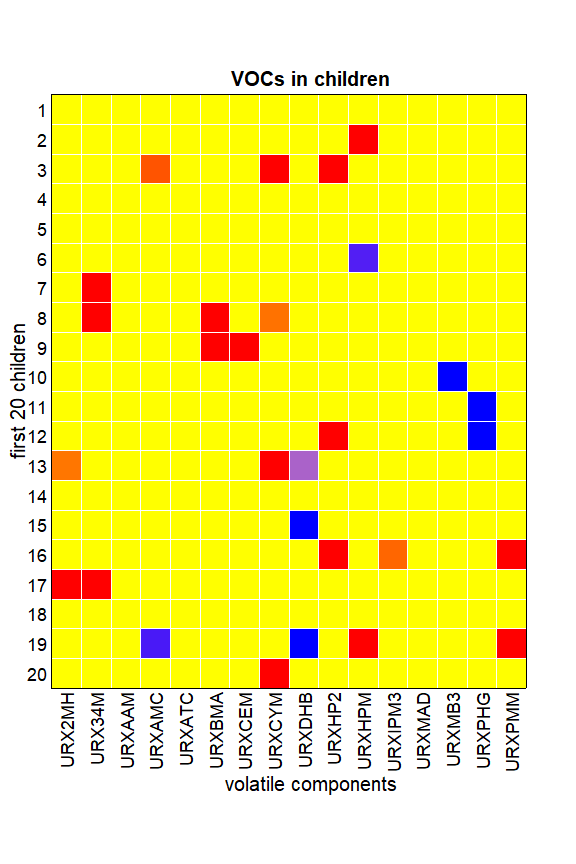``````# dev.off()
rm(rowsToShow)
``````

Now analyze the flagged cells and compare them with the cells found based on marginal outlyingness.

``````W <- matrix(0, nrow(X), ncol(X))
W[indcells] <- 1
# Variable 8 has a substantial number of red cells.
# Its total number of outlying cells:
sum(W[,8])/nrow(X)
``````
``````##  0.1171875
``````
``````# Variable 8 has 12% of outlying cells.

# Since quant = 0.99 these are the cells with absolute
# residual above sqrt(qchisq(p=0.99,df=1)) = 2.575829 .
# Variable 8 is "URXCYM":
#   N-Acetyl-S-(2-cyanoethyl)-L-cysteine (ng/mL)
# this is a well-known biomarker for exposure to tobacco
# smoke. see e.g.
# https://journals.plos.org/plosone/article?id=10.1371/journal.pone.0210104
# Adults who smoke usually have high values.

# How many URXCYM values in this set are marginally outlying?
# If we would use univariate outlier detection, few of
# the URXCYM values in this set would be considered suspicious:
meds = apply(X,2,FUN="median")
cutoff = sqrt(qchisq(p=0.99,df=1)); cutoff
``````
``````##  2.575829
``````
``````cellInd = which(abs(Zres[,8]) > cutoff)
length(cellInd)/nrow(X) # almost 12%
``````
``````##  0.1171875
``````
``````marginalInd = which(abs(Z[,8]) > cutoff)
length(marginalInd)/nrow(X) # under 2%
``````
``````##  0.01953125
``````
``````# Even for perfectly gaussian data this would already be 1%.

# pdf("ZresVersusZ.pdf",width=5.4,height=5.4) # sizes in inches
plot(Z[,8], Zres[,8], xlab = "",ylab = "",main = "",pch = 16,
col = "black", xlim=c(-3,5))
title(main="log(URXCYM) in children aged 10 or younger",
line=1) # , cex.lab=1.2, family="Calibri Light")
title(ylab="standardized cellwise residuals", line=2.3)
title(xlab="robustly standardized marginal values", line=2.3)
abline(h=cutoff, col="red")
abline(h=-cutoff, col="red")
abline(v=cutoff, col="red")
abline(v=-cutoff, col="red")
``````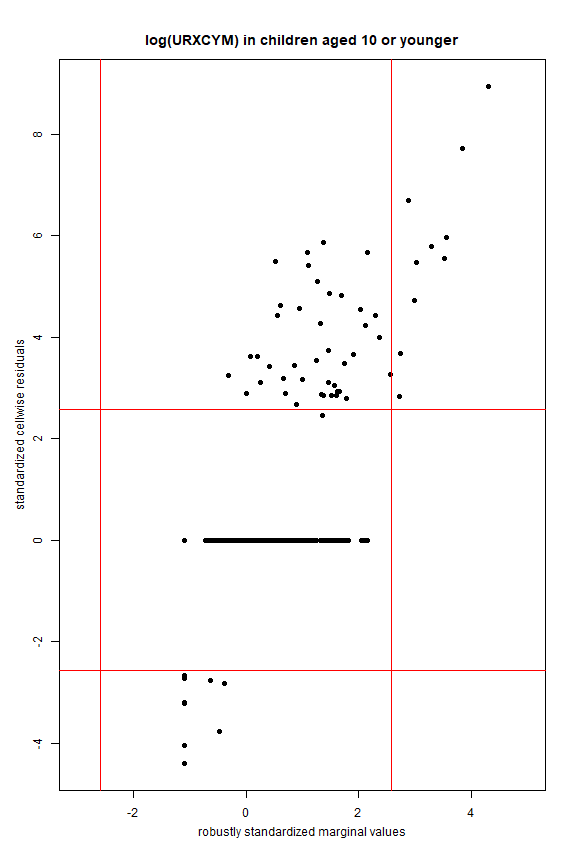``````# dev.off()
# Many outlying residuals occur at inlying marginal values.
# These persons' URXCYM is high relative to the other
# compounds (variables) in the same person.
``````

We now link the findings with the data on household smoking.

``````# Look at the residuals for the children who
# live together with people who smoke.
# We consider 4 categories:

# children without smokers in their family:
nonsmokers = which(data_VOC\$SMD460 == 0)
length(nonsmokers)
``````
``````##  340
``````
``````# at least one adult smokes, but not in the home:
noneInHome = which((data_VOC\$SMD460 > 0) &
(data_VOC\$SMD470 == 0))
length(noneInHome)
``````
``````##  109
``````
``````# children with 1 person smoking in their home:
oneInHome = which(data_VOC\$SMD470 == 1)
length(oneInHome)
``````
``````##  33
``````
``````# children with 2 people smoking in their home:
twoInHome = which(data_VOC\$SMD470 == 2)
length(twoInHome)
``````
``````##  11
``````
``````length(which(Zres[nonsmokers,8] > 0))/length(nonsmokers)
``````
``````##  0.05294118
``````
``````length(which(Zres[noneInHome,8] > 0))/length(noneInHome)
``````
``````##  0.1192661
``````
``````length(which(Zres[oneInHome,8] > 0))/length(oneInHome)
``````
``````##  0.3636364
``````
``````length(which(Zres[twoInHome,8] > 0))/length(twoInHome)
``````
``````##  0.7272727
``````
``````# So 36% of the children living in a house with one smoker
# have suspiciously high levels for this biomarker.
# 73% of the children living in a house with two smokers
# have suspiciously high levels for this biomarker.

cellMap(X, Zres,
indcells = which(W == 1),
columnlabels = colnames(X),
showrows = oneInHome,
rowlabels = 1:512,
mTitle = "VOCs in children",
rowtitle = "",
columntitle = "volatile components",
sizetitles = 2,
drawCircles = F)
``````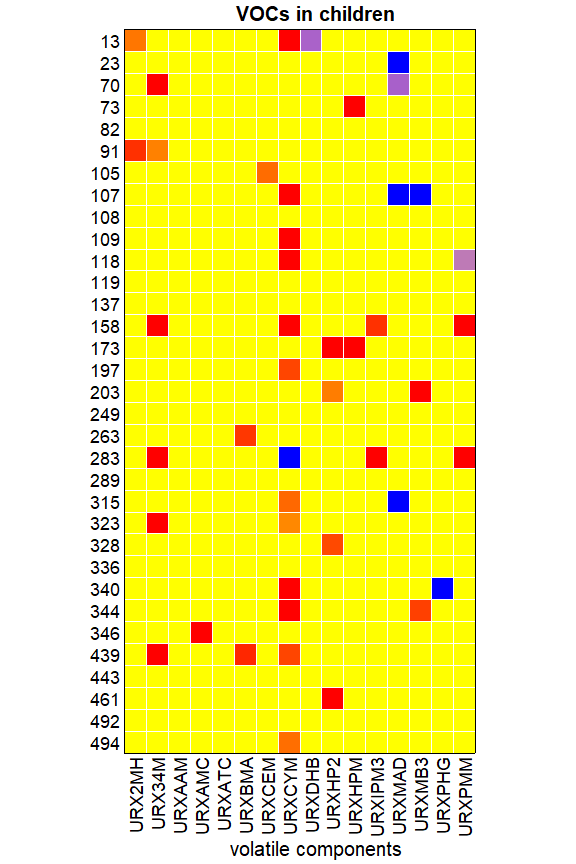``````cellMap(X, Zres,
indcells = which(W == 1),
columnlabels = colnames(X),
showrows = twoInHome,
rowlabels = 1:512,
mTitle = "VOCs in children",
rowtitle = "",
columntitle = "volatile components",
sizetitles = 2,
drawCircles = F)
``````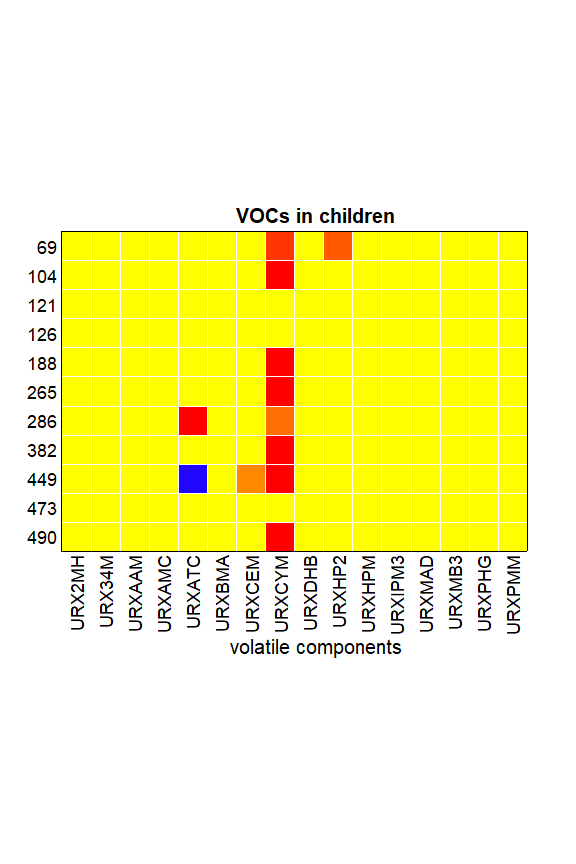``````# For one or more smokers in the house:
smokeInHome = c(oneInHome,twoInHome)
length(smokeInHome)
``````
``````##  44
``````
``````cellMap(X, Zres,
indcells = which(W == 1),
columnlabels = colnames(X),
showrows = smokeInHome,
rowlabels = 1:512,
mTitle = "VOCs in children",
rowtitle = "",
columntitle = "volatile components",
sizetitles = 2,
drawCircles = F)
``````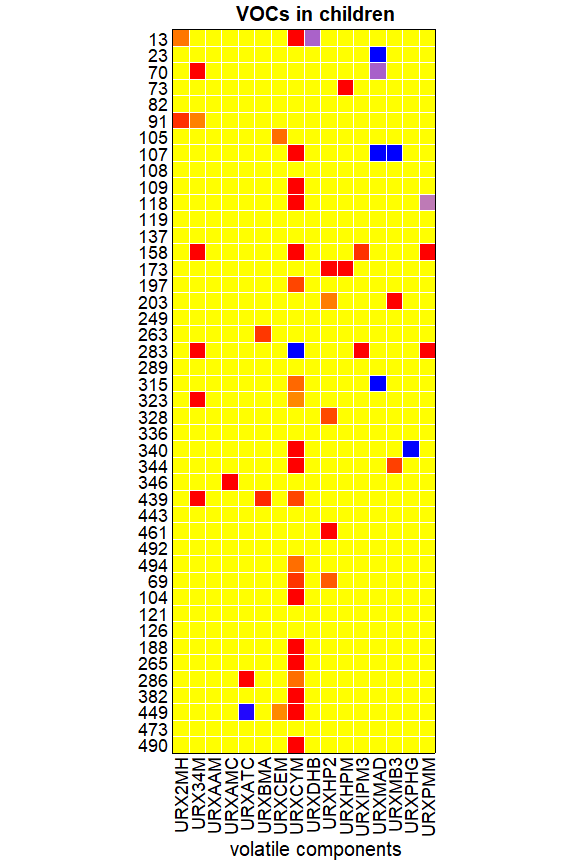``````# In all of these cellmaps the variable URXCYM stands out!

# If we would use a univariate detection bound, many of these values
# wouldn't be considered suspicious:

length(which(Z[nonsmokers] > cutoff))/length(nonsmokers)
``````
``````##  0.02058824
``````
``````length(which(Z[noneInHome] > cutoff))/length(noneInHome)
``````
``````##  0.02752294
``````
``````length(which(Z[oneInHome] > cutoff))/length(oneInHome)
``````
``````##  0
``````
``````length(which(Z[twoInHome] > cutoff))/length(twoInHome)
``````
``````##  0
``````
``````# Here the fractions are not even increasing with the number of smokers.

plotdata = matrix(c(length(which(Zres[nonsmokers,8] > 0))/length(nonsmokers),
length(which(Zres[noneInHome,8] > 0))/length(noneInHome),
length(which(Zres[oneInHome,8] > 0))/length(oneInHome),
length(which(Zres[twoInHome,8] > 0))/length(twoInHome),
length(which(Z[nonsmokers] > cutoff))/length(nonsmokers),
length(which(Z[noneInHome] > cutoff))/length(noneInHome),
length(which(Z[oneInHome] > cutoff))/length(oneInHome),
length(which(Z[twoInHome] > cutoff))/length(twoInHome)),
nrow = 2, byrow = TRUE)

# pdf("cellwise_marginal.pdf",width=5.4,height=5.4)
matplot(1:4, t(plotdata), type = "b", pch = 16, lwd = 3,
cex = 2, xlab = "", xaxt = "n", ylab = "", yaxt = "n",
ylim = c(0, 0.8), col = c("blue", "red"), lty = 1)
axis(side = 1, labels = c("none", "0 in home", "1 in home", "2 in home"),
at = 1:4, cex.axis = 1.3)
axis(side = 2, labels = seq(0, 100, by = 20),
at = seq(0, 1, by = 0.2), cex.axis = 1.3)
legend("topleft", fill = c("blue", "red"),
legend = c("cell residuals","marginal values"), cex = 1.3)
title(main="Effect of smokers on elevated URXCYM in children",
line=1.2)
title(ylab="% of children with elevated URXCYM",cex.lab=1.3, line=2.3)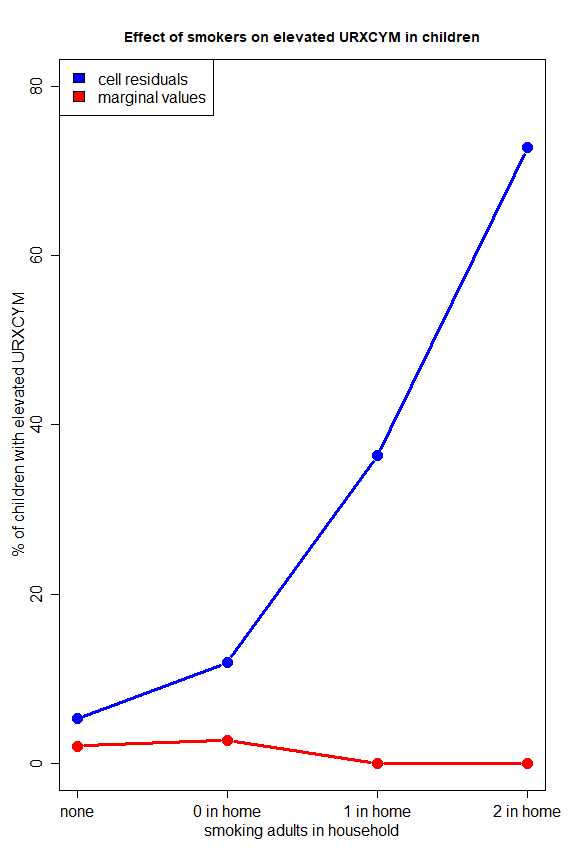``````# dev.off()